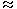# table chart aptitude questions

Study the following table and answer the questions based on it.

Number of Candidates Appeared, Qualified and Selected in a Competitive Examination from Five States Delhi, H.P, U.P, Punjab and Haryana Over the Years 1994 to 1998

 Year Delhi H.P U.P Punjab Haryana App Qual Sel App Qual Sel App Qual Sel App Qual Sel App Qual Sel 1997 8000 850 94 7800 810 82 7500 720 78 8200 680 85 6400 700 75 1998 4800 500 48 7500 800 65 5600 620 85 6800 600 70 7100 650 75 1999 7500 640 82 7400 560 70 4800 400 48 6500 525 65 5200 350 55 2000 9500 850 90 8800 920 86 7000 650 70 7800 720 84 6400 540 60 2001 9000 800 70 7200 850 75 8500 950 80 5700 485 60 4500 600 75
1. For which state the average number of candidates selected over the years is the maximum?
 A. Delhi B. H.P C. U.P D. Punjab

Explanation:

The average number of candidates selected over the given period for various states are:

 For Delhi = 94 + 48 + 82 + 90 + 70 = 384 = 76.8. 5 5

 For H.P. = 82 + 65 + 70 + 86 + 75 = 378 = 75.6. 5 5

 For U.P. = 78 + 85 + 48 + 70 + 80 = 361 = 72.2. 5 5

 For Punjab = 85 + 70 + 65 + 84 + 60 = 364 = 72.8. 5 5

 For Haryana = 75 + 75 + 55 + 60 + 75 = 340 = 68. 5 5

Clearly, this average is maximum for Delhi.

2. The percentage of candidates qualified from Punjab over those appeared from Punjab is highestin the year?
 A. 1997 B. 1998 C. 1999 D. 2000

Explanation:

The percentages of candidates qualified from Punjab over those appeared from Punjab during different years are:

 For 1997 =680 x 100% = 8.29%. 8200

 For 1998 =600 x 100% = 8.82%. 6800

 For 1999 =525 x 100% = 8.08%. 6500

 For 2000 =720 x 100% = 9.23%. 7800

 For 2001 =485 x 100% = 8.51%. 5700

Clearly, this percentage is highest for the year 2000.

3. In the year 1997, which state had the lowest percentage of candidates selected over the candidates appeared?
 A. Delhi B. H.P C. U.P D. Punjab

Explanation:

The percentages of candidates selected over the candidates appeared in 1997, for various states are:

 (i) For Delhi =94 x 100% = 1.175%. 8000

 (ii) For H.P. =82 x 100% = 1.051%. 7800

 (iii) For U.P. =78 x 100% = 1.040%. 7500

 (iv) For Punjab85 x 100% = 1.037%. 8200

 (v) For Haryana75 x 100% = 1.172%. 6400

Clearly, this percentage is lowest for Punjab.

4. The number of candidates selected from Haryana during the period under review is approximately what percent of the number selected from Delhi during this period?
 A. 79.5% B. 81% C. 84.5% D. 88.5%

Explanation:

Required percentage
 =(75 + 75 + 55 + 60 + 75) x 100% (94 + 48 + 82 + 90 + 70)
 =340 x 100% 384
= 88.54%88.5%

5. The percentage of candidates selected from U.P over those qualified from U.P is highest in the year?
 A. 1997 B. 1998 C. 1999 D. 2001

Explanation:

The percentages of candidates selected from U.P. over those qualified from U.P. during different years are:

 For 1997 =78 x 100% = 10.83%. 720

 For 1998 =85 x 100% = 13.71%. 620

 For 1999 =48 x 100% = 12%. 400

 For 2000 =70 x 100% = 10.77%. 650

 For 2001 =80 x 100% = 8.42%. 950

Clearly, this percentage is highest for the year 1998.